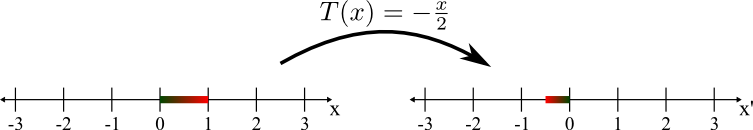# Math Insight

### Image: Compressing and reflecting by the linear transformation T(x)=-x/2The one-dimensional linear transformation $T(x)=-1/2$ maps the interval $[0,1]$ to the interval $[-1/2,0]$. The mapping compressed the interval into a new interval of one-half the original length. it also reflected the interval, so that part of the interval that was originally on the right is mapped into the left side of the transformed interval. The red to green gradient on the intervals illustrates the mapping, as each point on the interval is mapped a point of the same color.

Image file: linear_transformation_minus_half_x.png

Source image file: linear_transformation_minus_half_x.svg
Source image type: Inkscape SVG